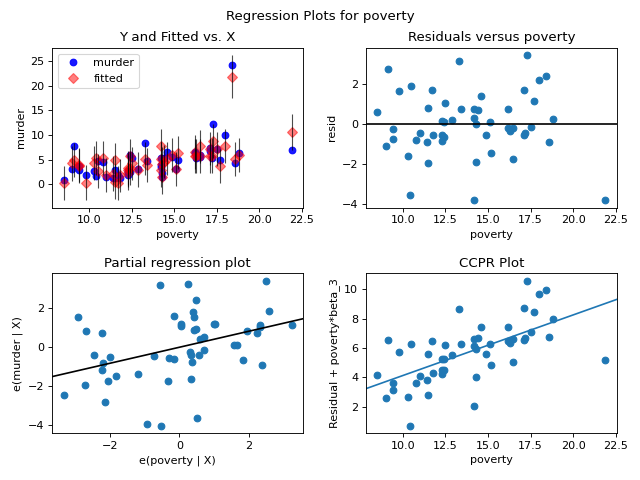statsmodels.graphics.regressionplots.plot_regress_exog¶

statsmodels.graphics.regressionplots.plot_regress_exog(results, exog_idx, fig=None)[source]

Plot regression results against one regressor.

This plots four graphs in a 2 by 2 figure: ‘endog versus exog’, ‘residuals versus exog’, ‘fitted versus exog’ and ‘fitted plus residual versus exog’

Parameters
resultsresult instance

result instance with resid, model.endog and model.exog as attributes

exog_idxint or str

Name or index of regressor in exog matrix

figMatplotlib figure instance, optional

If given, this figure is simply returned. Otherwise a new figure is created.

Returns
figmatplotlib figure instance

Examples

Load the Statewide Crime data set and build a model with regressors including the rate of high school graduation (hs_grad), population in urban areas (urban), households below poverty line (poverty), and single person households (single). Outcome variable is the muder rate (murder).

Build a 2 by 2 figure based on poverty showing fitted versus actual murder rate, residuals versus the poverty rate, partial regression plot of poverty, and CCPR plot for poverty rate.

>>> import statsmodels.api as sm
>>> import matplotlib.pyplot as plot
>>> import statsmodels.formula.api as smf

>>> fig = plt.figure(figsize=(8, 6))